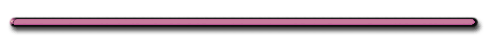HomeDescribe the Motion Below

Velocity? / Acceleration?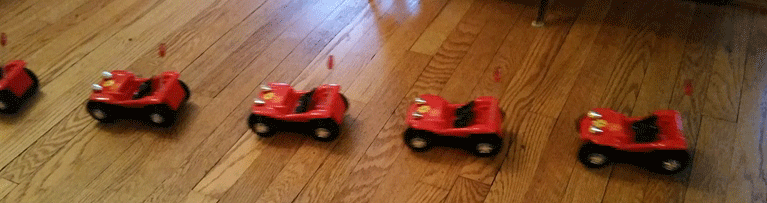Amusing Surface Tension Experiment

Video Instruction

showmethephysics.com

V. Finding displacement using V vs t graphs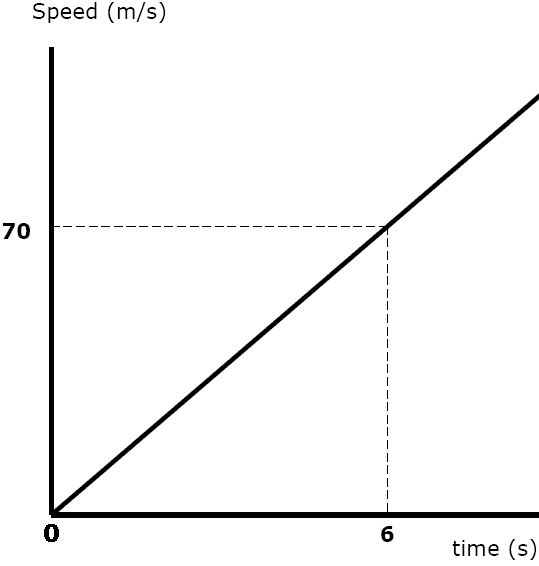since V = Δd/t

then Δd = Vt

=

Area under the plot

= displacement (distance)Ex) What is the displacement this object covered from t = 0 to t = 6 seconds?d = Area of the triangle:

= 1/2bh

= 1/2(6 sec)(70m/sec)

= 210 m

Ex 1)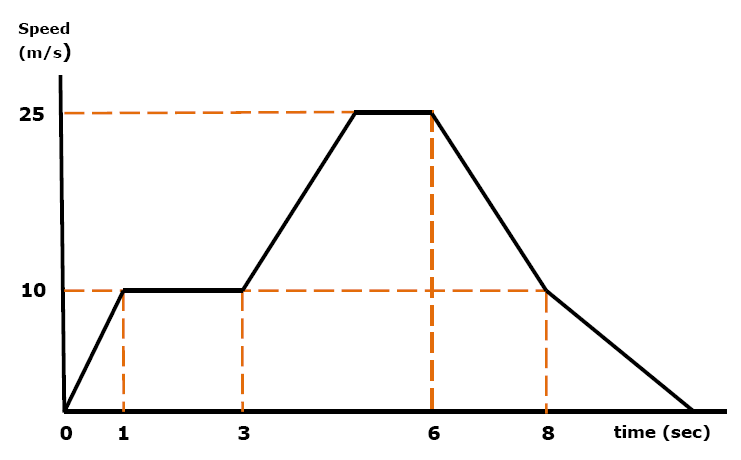Object's displacement from
t = 1 sec to t = 3 sec?d = Area = base x height

= (2 sec)(10 m/sec) = 20 m

Ex 2) Object's displacement from t = 6 sec to t = 8 sec ?d = area of trapezoid

= 1/2 (h1 + h2) x based = (25 m/s + 10 m/s) (2 sec) 2

= 35 mSmallest displacement?

Show Me The PhysicsLargest B, Smallest D

AP Question

Ex 3)

a) Find displacement after 4 seconds

b) At what time does the object return to its starting point?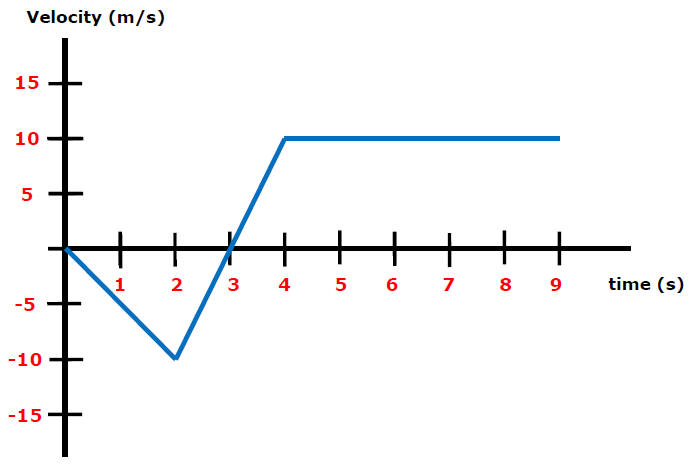After 4 seconds:

1/2(3 sec)-10 m/s + 1/2(1 sec)10 m/s

-15 m + 5 m

= -10 m

Answer: 5 seconds when d = 0Ex 4)

When did car A catch up to car B?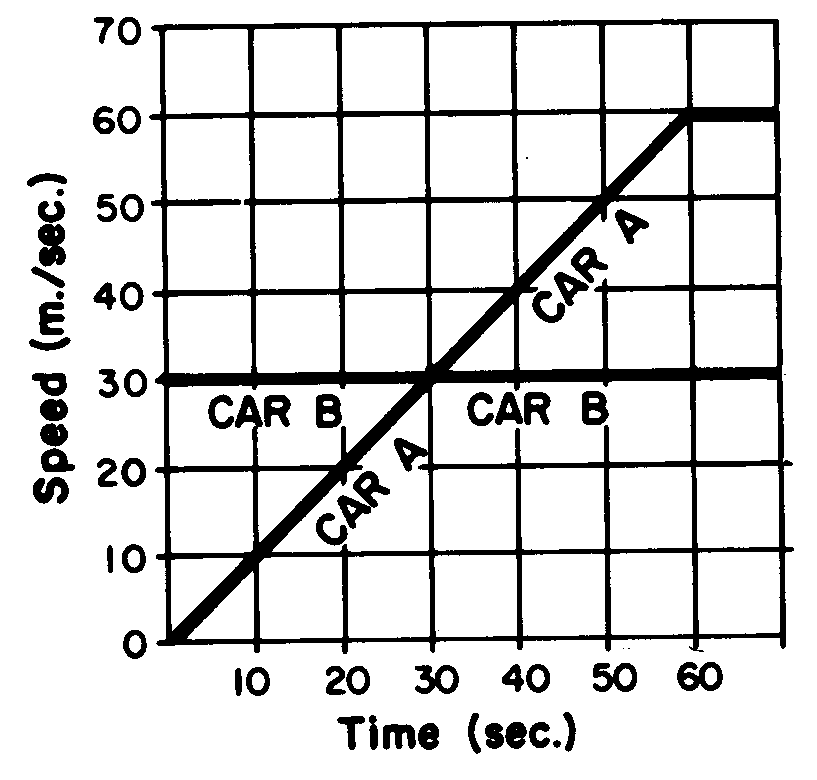Car A caught up to car B when their d are equal

At 60 sec:

Car  B: d= 60 m/s(30 sec) = 180 m

Car A: d =1/2(60 m/s)(60 sec) = 180 mFree Fall